# Solve system of linear equations by Cramer's rule onlineOne of the ways to solve the system of the linear algebraic equations (SLAE) is to use the Cramer's rule . Suppose we have the SLAE: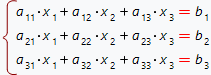To solve it, one need to find such the values of the variables x1, x2, x3 which convert the initial SLAE to the correct identity. To show how the Cramer's rule works rewrite our initial SLAE in the matrix form: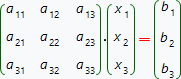First step of the Cramer's rule, is to test the value of the determinant of the SLAE matrix: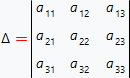If the computed determinant doesn't equal to zero, then the initial SLAE has the single solution, which can be found by the Cramer's rule. If the computed determinant does equal to zero, then the initial SLAE may either have no solution or have the inifinity set of the solutions which cannot be found by the Cramer's rule.

Suppose, the computed determinant doesn't equal to zero: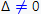then, by the Cramer's rule, the solution of the SLAE can be found by using the formulas: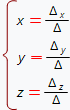here, x, y and z are the determinants derived from the determinant by changing the corresponding column to the vector of the free coefficients. For example, the determinant x received from the determinant by changing the first column to the vector of the free coefficients: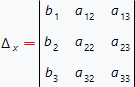By using this method one can receive the determinants y and z. It should be noted, that the Cramer's rule is applicable to the SLAEs where the number of the equations equal to the number of the variables.

Our online calculator solves the SLAE by the Cramer's rule with step by step solution. SLAE coefficients can be not only numbers of fractions but also the parameters. To use the calculator, one need to input the SLAE and choose the SLAE's variables to find.

Solve slae by Cramer's rule
,,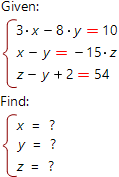System of linear algebraic equations you want to solve: